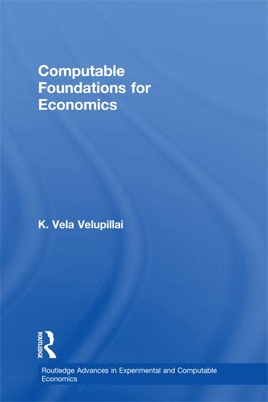• USD 46.99

## Descripción de editorial

Computable Foundations for Economics is a unified collection of essays, some of which are published here for the first time and all of which have been updated for this book, on an approach to economic theory from the point of view of algorithmic mathematics. By algorithmic mathematics the author means computability theory and constructive mathematics. This is in contrast to orthodox mathematical economics and game theory, which are formalised with the mathematics of real analysis, underpinned by what is called the ZFC formalism, i.e., set theory with the axiom of choice. This reliance on ordinary real analysis and the ZFC system makes economic theory in its current mathematical mode completely non-algorithmic, which means it is numerically meaningless.

The book provides a systematic attempt to dissect and expose the non-algorithmic content of orthodox mathematical economics and game theory and suggests a reformalization on the basis of a strictly rigorous algorithmic mathematics. This removes the current schizophrenia in mathematical economics and game theory, where theory is entirely divorced from algorithmic applicability – for experimental and computational exercises.

The chapters demonstrate the uncomputability and non-constructivity of core areas of general equilibrium theory, game theory and recursive macroeconomics. The book also provides a fresh look at the kind of behavioural economics that lies behind Herbert Simon’s work, and resurrects a role for the noble classical traditions of induction and verification, viewed and formalised, now, algorithmically. It will therefore be of particular interest to postgraduate students and researchers in algorithmic economics, game theory and classical behavioural economics.